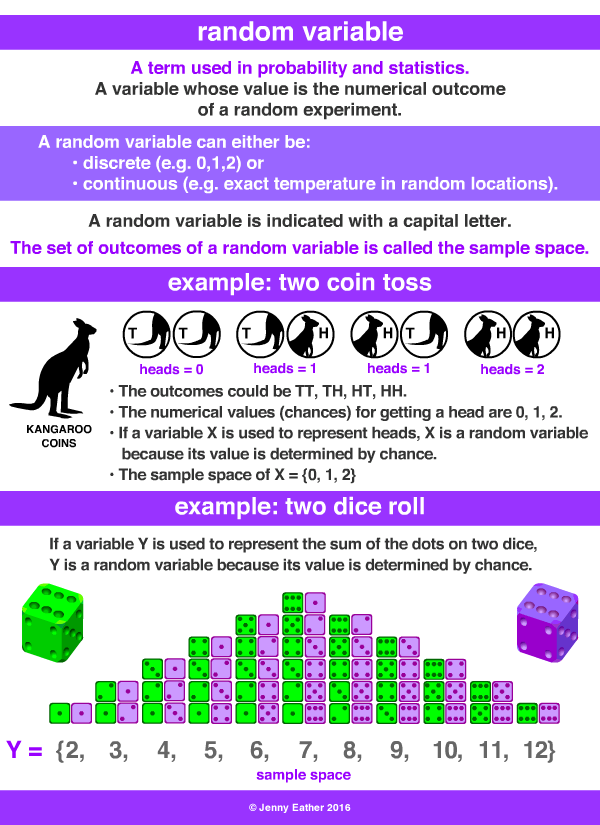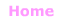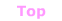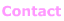Aa Bb Cc Dd Ee Ff Gg Hh Ii Jj Kk Ll Mm Nn Oo Pp Qq Rr Ss Tt Uu Vv Ww Xx Yy Zz

Rr

random variable

• a variable whose value is the numerical outcome
of a probability experiment.

It is random because its value is
determined by chance.

• the set of outcomes of a random variable
is called the sample space.
• a random variable may have either discrete (exact) values
or continuous (infinite) values.
• indicated by a capital letter ... to distinguish it
from an algebraic variable.

EXAMPLES:© Jenny Eather 2014. All rights reserved.